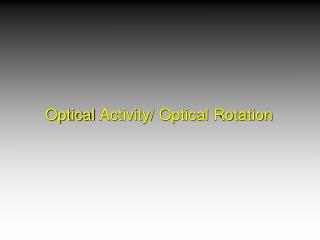DownloadDownload PresentationOptical Activity/ Optical Rotation

# Optical Activity/ Optical Rotation

Télécharger la présentation## Optical Activity/ Optical Rotation

- - - - - - - - - - - - - - - - - - - - - - - - - - - E N D - - - - - - - - - - - - - - - - - - - - - - - - - - -
##### Presentation Transcript

1. Optical Activity/ Optical Rotation

2. Optical Activity

3. Optical Activity A substance is optically active if it rotates a plane of polarized light. In order for a substance to exhibit opticalactivity, it must be chiral and one enantiomermust be present in excess of the other.

4. Polarimetry http://chemconnections.org/organic/chem226/226assign-12.html#polarimetry http://chemconnections.org/organic/chem226/Labs/opt-rotation/opt-rot-I.html

5. Light: electromagnetic radiation Optical activity is usually measured using light having a wavelength of 589 nm; this is the wavelength of the yellow light from a sodium lamp and is called the D line of sodium.

6. Optical Activity Counterclockwise (-); l Clockwise (+); d Different from absolute (R,S) configuration

7. Optical Activity/ Rotation • The angular rotation observed in a polarimeter depends on: • the optical activity of the compound • the concentration of the sample • the path length of the sample cell • A compound’s specific rotation [a] can be used as a characteristic physical property of a compound: • the rotation observed using a 10-cm sample cell and a concentration of 1 g/mL.

8. Optical Activity [a]= specific rotation c = concentration in g/mL l = path length in dm a (observed) = rotation observed for a specific sample

9. Optical Activity Example: A solution of 2.0 g of (+)-glyceraldehyde in 10.0 mL of water was placed in a 100. mm polarimeter tube. Using the sodium D line, a rotation of 1.74o was observed at 25oC. Calculate the specific rotation of (+)-glyceraldehyde.

10. Optical Activity Given:a (obs) = 1.74o Find: [a]

11. Question:Calculate []D • A 1.00-g sample is dissolved in 20.0 mL ethanol. 5.00 mL of this solution is placed in a 20.0-cm polarimeter tube at 25C. The observed rotation is 1.25 counterclockwise. []D = A) -1.25  B) -6.125  C)-12.5  D) +61.25 

12. Racemic mixture A 50:50 mixture containing equal quantities of enantiomers is called a racemicmixture. A racemic mixture is optically inactive. (a = 0) Asample that is optically inactive can beeither an achiral substance or a racemicmixture.

13. Optical purity an optically pure substance consists exclusively of a single enantiomer enantiomeric excess = % one enantiomer – % other enantiomer % optical purity = enantiomeric excess

14. Some mixtures are neither optically pure (all one enantiomer) nor racemic (equal mixture of both enantiomers). They can be defined by their optical purity which equals enantiomeric excess. observed specific rotation optical purity = specific rotation of the pure enantiomer

15. Optical Activity Example: (-)-2-butanol has a specific rotation of - 13.5o while the specific rotation of (+)-2-butanol is +13.5o. Calculate the optical purity of a mixture containing (+) and (-)-2-butanol if the mixture has an observed rotation of – 8.55o. Does the mixture contain more (+) or more (-)-2-butanol? o.p. = -8.55ox 100% = 63.3% -13.5o

16. Optical Activity • Enantiomeric excess. • Numerically identical to optical purity e.e. = o.p. = excess of one over the other x 100% entire mixture

17. Optical Activity What is the e.e of a mixture containing 25% (+)-2-butanol and 75% (-)-2-butanol if the specific rotation of (+)-2-butanol is 13.5o.

18. Question A sample of a pure R-enantiomer has a specific rotation of – 40°. A mixture of R-/S- enantiomers has an observed optical rotation of + 22°. What is the % ee of the mixture? A. 55 % eeR B. 55 % eeS C. 18 % eeR D. 0.55 % eeRE. none of the above

19. Question If a sample is 50 % ee of R stereoisomer, what is the % R in the mixture? A. 50B. 100C. 25D. 75

20. Question If a chemical reaction produces a mixture of 80% R and 20 % S, what is the % ee? 80 60 20 10 4

21. Question If a sample of the pure Renantiomer of a molecule has a specific rotation of – 40° what is the specific rotation of pure S? –60° +60° –40° +40° cannot be determined

22. Question If the specific rotation of (R)-2-methylhexan-2-ol is –35°, what is the specific rotation of (S)-hexan-2-ol? +35° –35° It is negative but value cannot be determined. It is positive but value cannot be determined. It cannot be determined.

23. Question If a sample of the pure Renantiomer of a molecule has a specific rotation of – 40° and an enantiomeric mixture of that molecule has an 82% eeS, whatshoulld be the observed specific rotation of the sample? +40° +8.2° +32.8° –48.7° cannot be determined

24. Question Calculate the relative proportions of (+)-2-butanol, []D = +13.5o, and (-)-2-butanol, []D = -13.5o, required to give a specific rotation of +0.45o. 50% R : 50% S 90% R : 10% S 3.3 % R : 92.7% S 52% R : 48% S cannot be determined